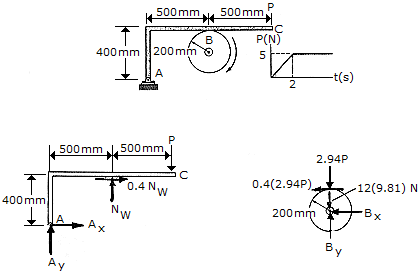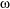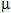Engineering Mechanics - PKRB: Impulse and Momentum - Discussion

Discussion :: PKRB: Impulse and Momentum - General Questions (Q.No.5)

5.The 12-kg disk has an angular velocity of= 20 rad/s. If the brake ABC is applied such that the magnitude of force P varies with time as shown, determine the time needed to stop the disk. The coefficient of friction at B is= 0.4.

 [A]. t = 13.00 s [B]. t = 7.00 s [C]. t = 8.92 s [D]. t = 5.08 s

Explanation:

No answer description available for this question.

 Kalidass said: (Jul 31, 2016) I want derivation part of the anwser.